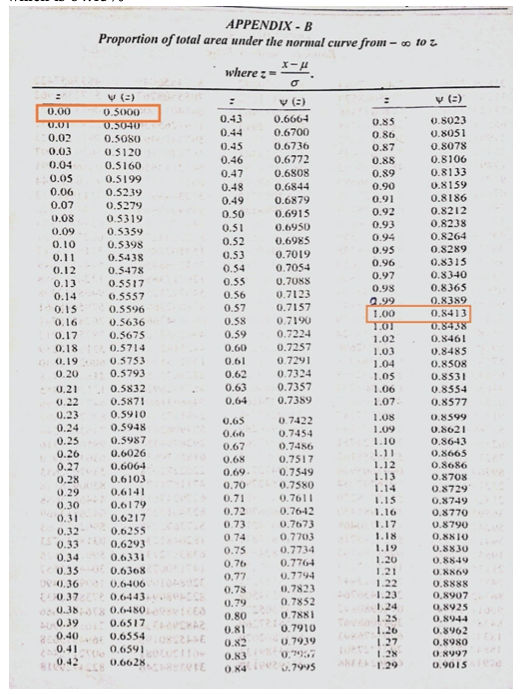# Numerical on PERT (Program Evaluation and Review Technique)

### For the given activities determine:1. Critical path using PERT.2. Calculate variance and standard deviation for each activity.3. Calculate the probability of completing the project in 26 days.

Activity t o t m t p
1-2 6 9 12
1-3 3 4 11
2-4 2 5 14
3-4 4 6 8
3-5 1 1.5 5
2-6 5 6 7
4-6 7 8 15
5-6 1 2 3

Solution: First of all draw the network diagram for given data as shown below: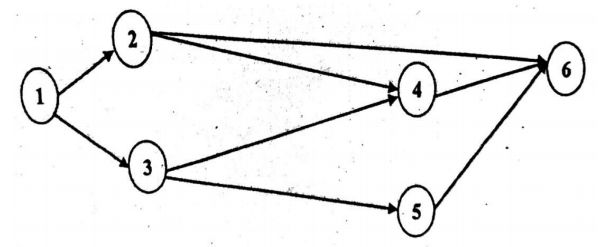Here the time for completion of activities are probabilistic. So, using given values of time we will find the expected time to completion the activities and variance.

$\text {Expected time } \quad t_e = {t_o + 4t_m + t_p \over 6}$ $\text {Variance } V = \Big({t_p - t_o \over 6}\Big)^2$

For each given activity we will calculate the expected time as follows:

Activity t o t m t p $t_e = {t_o + 4t_m + t_p \over 6}$ Variance (V)
1-2 6 9 12 ${6+4 \times 9+12 \over 6} = 9$ $({12-6 \over 6})^2 = 1.000$
1-3 3 4 11 5 1.778
2-4 2 5 14 6 4.000
3-4 4 6 8 6 0.444
3-5 1 1.5 5 2 0.444
2-6 5 6 7 6 0.111
4-6 7 8 15 9 1.778
5-6 1 2 3 2 0.111

Now based on estimate time, we calculate the EST, EFT, LST and LFT for each activity to find out critical path of project as shown below. (Click here to know about calculation of EST, EFT, LST and LFT from CPM numerical)

Activity Duration EST EFT LST LFT Total Float
1-2 9 0 9 0 9 0
1-3 5 0 5 4 9 4
2-4 6 9 15 9 15 0
3-4 6 5 11 9 15 4
3-5 2 5 7 20 22 15
2-6 6 9 15 18 24 9
4-6 9 15 24 15 24 0
5-6 2 7 9 22 24 15Here the critical path is along the activities 1-2, 2-4, 4-6. So the critical path is 1-2-4-6. Following diagram is prepared to show critical path along with EST and LFT.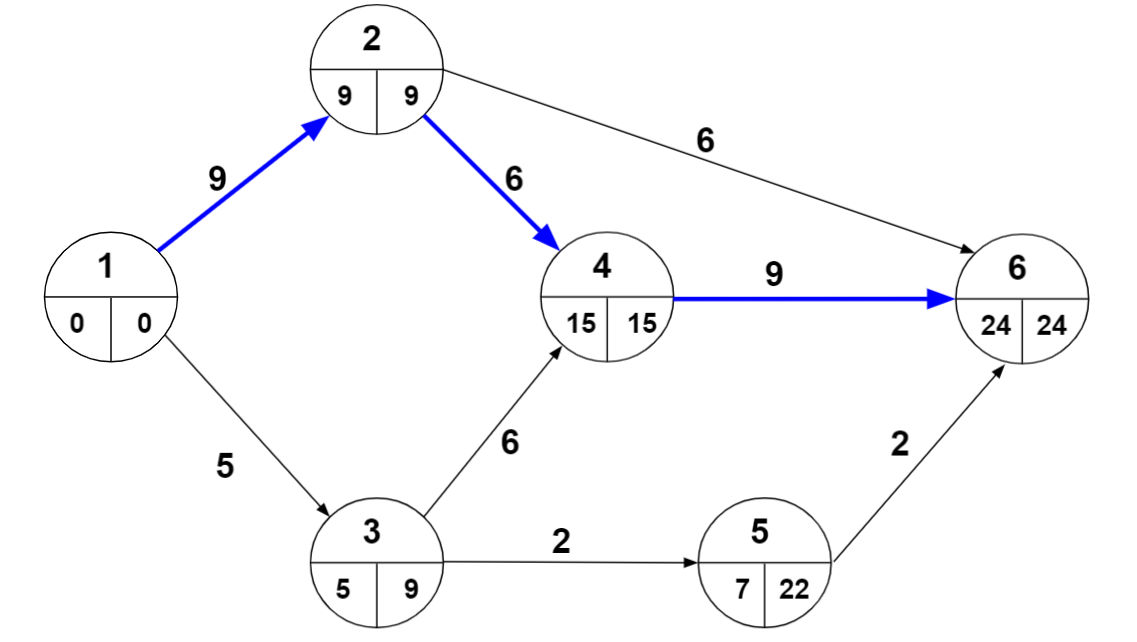$\therefore$ The critical path = 1-2-4-6 with time duration $(t_{cp})$ of 24 days.

Here standard deviation is calculated for activities of critical path. So we get

\begin{aligned} \sigma&= \sqrt{V_1 + V_2 + V_3} \\ \sigma&= \sqrt{1 + 4 + 1.778} \\ &= 2.6034 \end{aligned}

Now the probability of completion of project in that given time (t) of 26 days, can be calculate by below formula,

$Z = {t - t_{cp} \over \sigma} = {26 - 24 \over 2.6034} = 0.7682$

Using table in Appendix-B, we get probability $= 77.8 \%$

As you can see below in Appendix-B the first column Z that is the probability we find out in the example through formula. The second column $\psi(z)$ represent the probability in percentage $(\%)$.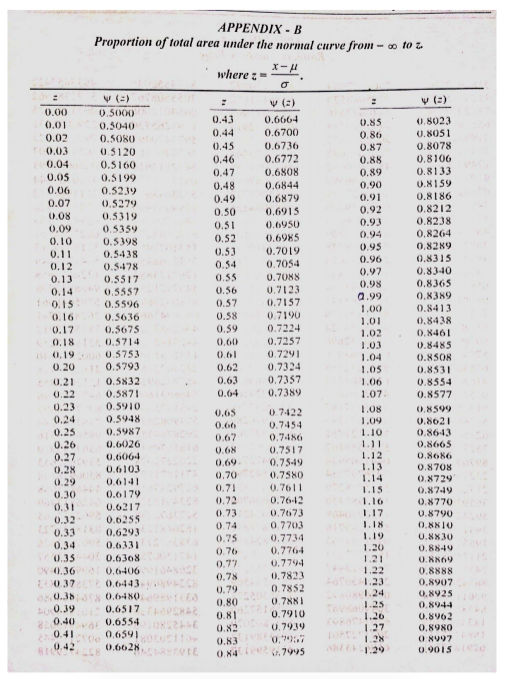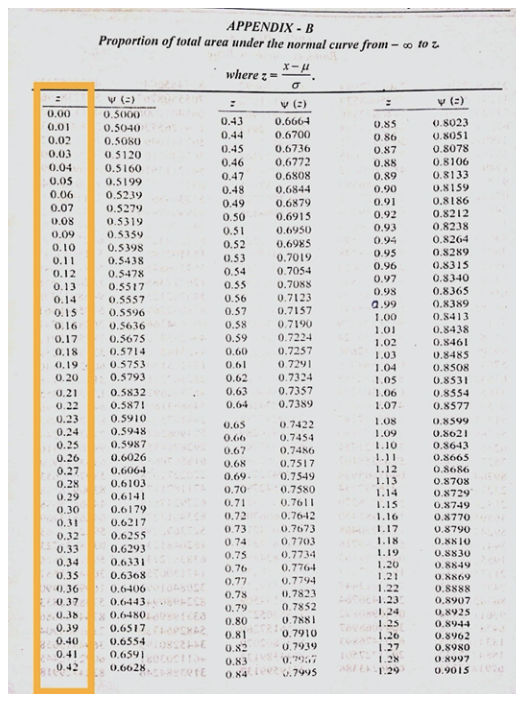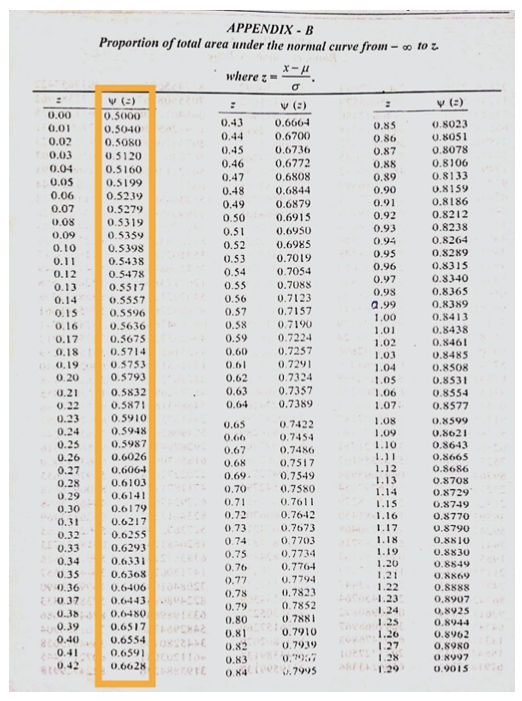As we have value of $Z=0.7682$ now you can see in table we have $Z=0.76$ which has $\psi(z)=0.7764$ and $Z=0.77$ has $\psi(z)=0.7794$ we take average of both we get $\psi(z)=0.7779=77.8\%$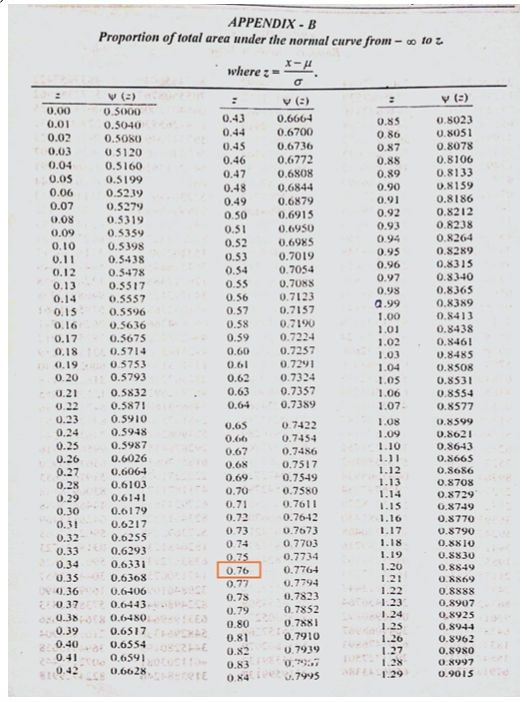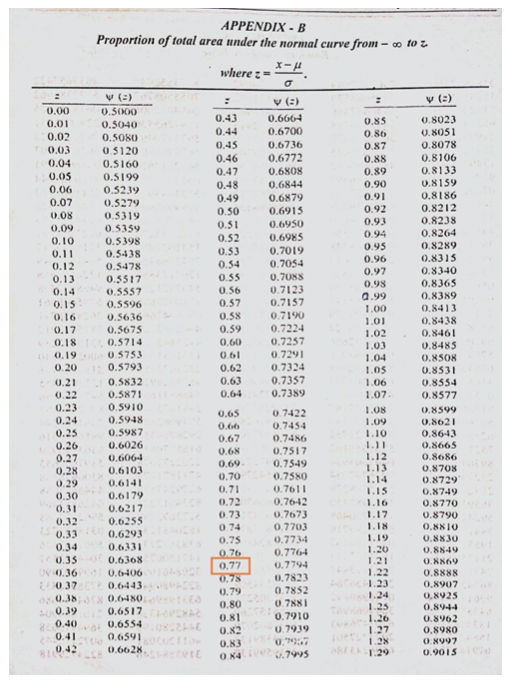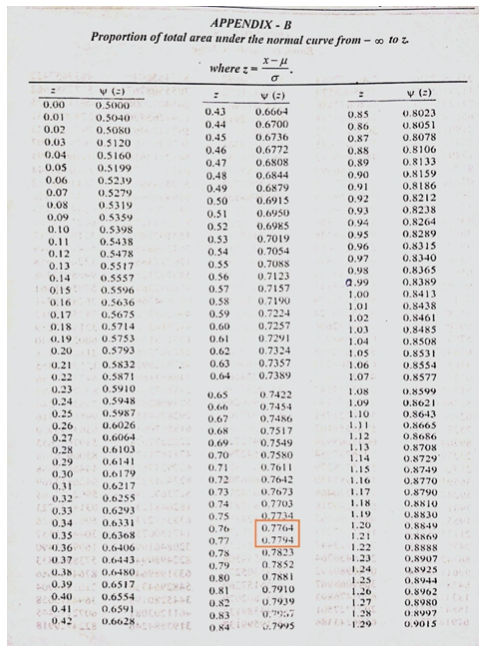As you can see for $Z=0 \space \space$ $\psi(z)=0.5000 \space \space$ which is $50\%$ and for $Z=1 \space \space$ $\psi(z)=0.8413 \space \space$ which is $84.13\%$